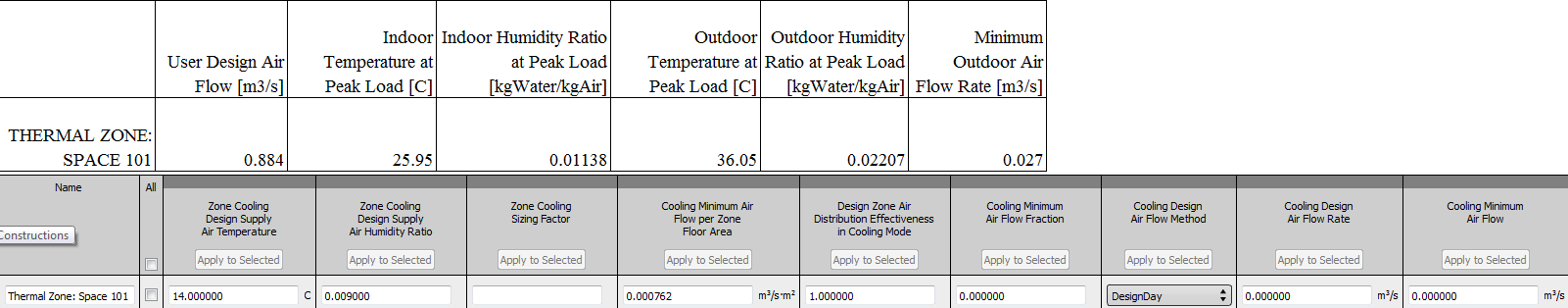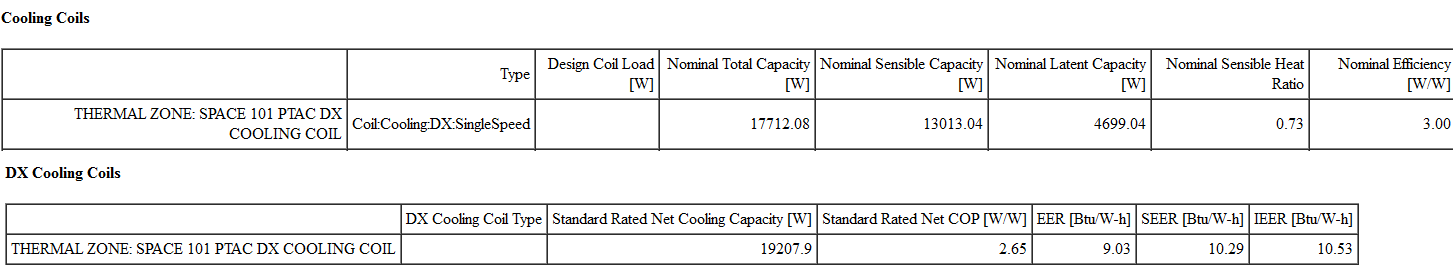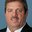Question-and-Answer Resource for the Building Energy Modeling Community
Get started with the Help page

# Coil sizing check in E+Hello, i would like to manually verify DX coil sizing in zonal equipment. The zone coil load should include ventilation and infiltration latent load. But unfortunately E+ standard report doesn't have them in desired format (g/kg instead of W). Update from Mr. Rraustad suggestion and E+ documentation : input as follow :Bases on psychrometric coil sizing formula :

set  standard air density (1,2 kg/ m3)


mixed air flow = User Calc air flow =0.884 m3/s = 0.857(return air flow) +0.027 (outdoor air flow)

Enthalpy outdoor (36oC, 0.02207g/kg) = 92.87 kJ/kg ;Enthalpy return air/room air (26oC, 0.01138 g/kg) = 55.21 kJ/kg

Enthalpy supply air (14oC, 0.009 g/kg) = 36.87 kJ/kg

Enthalpy mix = ( Outdoor air enthalpy * Outdoor air flow + Return air flow * Return outdoor enthalpy)/mixed air flow = 56.37 kJ/kg -> air temperature = 26.3 oC air density = 1.157 kg/m3

Performance CAPFT : x = mixed air wetbulb = 19.68 oC , y = outdoor drybulb = 36 oC

CapFT= 1.5509 + (-0.07505) * 19.68 + 0.0031 * 19.68^2 + 0.0024 * 36 + (0.00005) * 36^2 + 36 * 19.68 *(-0.00043) = 1.121

Coil capacity =  (Enthalpy mix - Enthalpy sizing zone ) * (air density) *  (airflow) /  (CapFT)
=     (55.21 - 36.87) * 1.157 * 0.884 /1.121 = 17782 W


The coil report for PTAC :Great Thanks to Mr Rraustad for validation.

edit retag close merge delete

i look at component sizing summary and equipment summary reports. i focus on basic sizing calculations of local system like PTHP, PTAC, VAV box,...

I stepped through the PTHP example file last night and found a match to the DX cooling coil size. I'll have to repeat that tonight. Or if you send me your file, I'll validate your coil.

1

Your file: Mixed T = 26.27, w = 0.01171, WB = 19.71, H = 56.248, rho = 1.1566, flow = 0.8838 CapFT = 1.12193, SupplyH = 36.81, Q = (56248-36810) * 1.1566 * 0.8838 / 1.12193 = 17710.2 W compared to 17712.08 W (rounding)

Sort by » oldest newest most votedThe Component Sizing Summary and Equipment Summary in the table reports show the DX coil size as total capacity (sensible + latent) reported as W (or Btu/hr if requested). The zone load is reported as sensible energy to meet the zone thermostat set point temperature. Ventilation and Infiltration are included in the coil sizing if these OA components are included in the model. The coil airflow is sized to meet the zone sensible load, and then includes the DX coil capacity as a function of temperature performance curve to calculate the coil's design total capacity.

$$Q_{coil} = \frac {\rho V ({h_{in} - h_{out})}}{CapFT}$$

where:
$Q_{coil}$ = coil total cooling capacity (sensible + latent)
$\rho$ = coil inlet air density
V = zone air volume flow rate determined by sizing routine (see *zsz.csv)
$h_{in}$ = coil inlet air enthalpy at peak time including OA
$h_{out}$ = coil outlet air enthalpy as specified in Sizing:Zone object
CapFT = capacity as a function of temperature curve output at coil inlet air temperatures

Update based on comment: The HVAC Sizing Summary report will show the values for User Design Air Flow. The indoor temperature and humidity ratio shown here does not include outdoor air. If OA is used, calculate a mixed air inlet condition using the OA fraction (OA flow/user design flow). Then use the mixed air condition to calculate coil inlet air density and enthalpy. The CapFT value is determined by entering the Indoor/Outdoor Temperature, converted to wet-bulb temperature, at Peak Load into the performance curve to find the value used in the coil sizing model.

The OA included in the coil sizing equation is the OA mixed with return air at the outdoor air system mixing box. This mixing of OA impacts the coil inlet air conditions. Infiltration will show up in the zone indoor conditions reported in HVAC Sizing Summary.

more

Thank a lot for formula. But it's hard to determine all those input with standard report of E+ for zonal equip like PTAC. i got from HVAC sizing summary Cooling Sensible - User Design Load (W) - it's same as zone component load summary report for sensible peak load. But how to get coil total cooling capacity (sensible + latent) form that value? i divided Cooling Sensible - User Design Load (W) with Design Size Gross Rated Sensible Heat Ratio in Component sizing summary and it often 9 % less than Design Size Gross Rated Total Cooling Capacity in Component Sizing Summary report

I try to figure your suggestion for infiltration. In HVAC sizing summary has only

Indoor Temperature at Peak Load [C] Indoor Humidity Ratio at Peak Load [kgWater/kgAir]  Outdoor Temperature at Peak Load [C]    Outdoor Humidity Ratio at Peak Load [kgWater/kgAir] Minimum Outdoor Air Flow Rate [m3/s]    Heat Gain Rate from DOAS [W]


so infiltration load should be calculation as outdoor enthalpy minus indoor enthaply then multiply with design infiltration rate and schedule factor ?

If you are interested only in coil sizing, then infiltration will impact zone load and zone conditions. The values for indoor temperature and humidity ratio include the impact of infiltration and is therefore included in the sizing equation shown above.

in case indoor temperature and humidity include the impact infiltration, should i take the grand total latent value included infiltration latent load in Estimated Cooling Peak Load Components when checking total load for dehumidifying coil sizing ?

The infiltration load will be included in the reported coil total load since the coil entering air conditions will include the impact of infiltration.